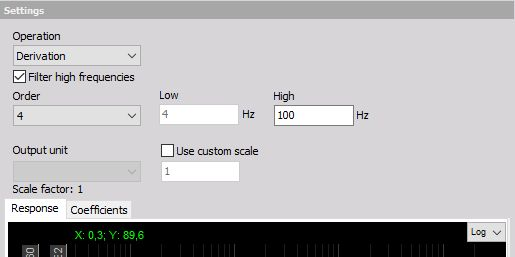# Derivation and double derivation

## Derivation

Derivation and double derivation is used, as the name already suggests, to calculation derivation of chosen input signals. Here the application range is not divided into two areas since the procedure is similar in all cases. The basic calculation is simple: we subtract current value from the previous one and divide by the time interval.

However, this might produce very noisy signals, especially with the high sampling frequency. We can look at the derivation as the 20 db / decade growing filter in the frequency domain. Sometimes it is therefore nice to cut the high-frequency contents. We can choose an option to Filter high frequencies. We choose the Order (at least 2 for derivation and at least 3 for double derivation) and FHigh - cutoff frequencies of the signal.

Let’s assume the example of calculating acceleration out of speed of the vehicle. With a car it is a fact that we can’t have the acceleration higher than for example 10 Hz coming from the real vehicle acceleration - higher values are basically vibrations.

So we can choose the 10 Hz as the FHigh high frequency and just get the real vehicle acceleration. Scale - scaling factor is similar, here the input is divided with second for derivation and divided with second squared for double derivation. So let’s calculate the scaling factor for example above:

1 km/hour / sec = 1000 m / 3600 sec /sec = 0.278 m/s2 = 0.278 / 9,81 g = 0.0283 g

We have to enter this value in the scale field to get correct results.